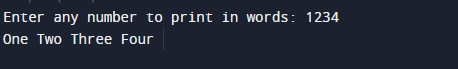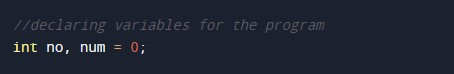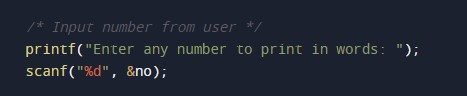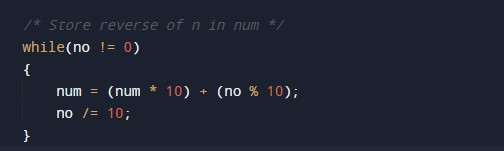C Program to Print Number in Words

In this tutorial you will learn about the C Program to Print Number in Words and its application with practical example.

C Program to Print Number in Words.

In this tutorial, we will learn to create a C program that will Print Numbers in Words in C programming.

Prerequisites

Before starting with this tutorial we assume that you are best aware of the following C programming topics:

• Operators in C Programming.
• Basic Input and Output function in C Programming.
• Basic C programming.
• While Loop in c programming.
• Switch Case in

Print Numbers in Words

As we all know the c programming is a very powerful language. In the c programming language, we have many pre-defined functions for arithmetic operations. but today we will take the input number and Print Number in Words with the help of the c programming language.

With the help of this program, we can Print Numbers in Words.

Output:-In the above program, we have first initialized the required variable.• no = it will hold the integer value of number input.
• num  = it will hold the integer value of number input.

Taking input number from the user.Program logic.Printing Output numbers.In this tutorial we have learn about the C Program to Print Number in Words and its application with practical example. I hope you will like this tutorial.